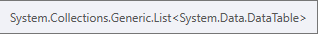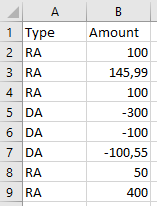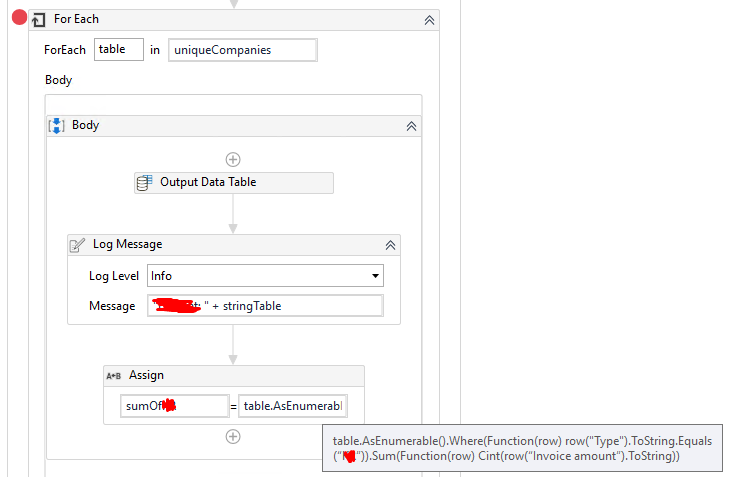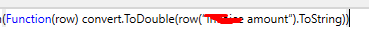# Sum of column where row is of a certain type

The input datable is of type :I want to store the sum of RA and DA in a two variables.

Can someone help me with a Linq statement that calculates the sum of RA in this case “795,99” and sum of DA in this case “-500,55”.

Hi,

are you sure that the type is List? So do you need a query for all datatables in the list or just for only one?

regards, Gio

Hi,

Its of type list because I use it in a For Each activity.
It’s dynamic and unique for each iteration.

Hi @atomic
to calculate the sum of RA use the below linq query

dt1.AsEnumerable().Where(Function(row) row(“Type”).ToString.Equals(“RA”)).Sum(Function(row) Cint(row(“Amount”).ToString))

use the same way for other values too apart from RA

Try this

Regards,

Nived N
Happy Automation

3 Likes

Hi @atomic

row(“Type”) should be put

I just edited my response

Getting this error when I run the activity:do the excel file contains ‘Type’ column ?

Maybe this helps:Maybe the problem is that its of type “List”?

1 Like

For each argument type should be DataTable type.

Please check with whether the column is in table or not

or try with row(0) instead of row(“Type”)

1 Like

can you try this @atomic,

outDt.AsEnumerable().Where(Function(x) (x(0).ToString.Equals(“RA”))).Sum(Function(r) Cint(r(1))).ToString

Cheers
@atomic

1 Like

My fault, forgot to change the name of the column.

I changed from Cint → convert.ToDouble but it works perfectly now.Thanks!

2 Likes

I will try this out also.

Thanks!

1 Like

This topic was automatically closed 3 days after the last reply. New replies are no longer allowed.